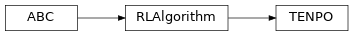# garage.tf.algos.te_npo¶

Natural Policy Optimization with Task Embeddings.

class TENPO(env_spec, policy, baseline, scope=None, discount=0.99, gae_lambda=1, center_adv=True, positive_adv=False, fixed_horizon=False, lr_clip_range=0.01, max_kl_step=0.01, optimizer=None, optimizer_args=None, policy_ent_coeff=0.0, encoder_ent_coeff=0.0, use_softplus_entropy=False, stop_ce_gradient=False, inference=None, inference_optimizer=None, inference_optimizer_args=None, inference_ce_coeff=0.0, name='NPOTaskEmbedding')Natural Policy Optimization with Task Embeddings.

See https://karolhausman.github.io/pdf/hausman17nips-ws2.pdf for algorithm reference.

Parameters
• env_spec (EnvSpec) – Environment specification.

• baseline (garage.tf.baselines.Baseline) – The baseline.

• scope (str) – Scope for identifying the algorithm. Must be specified if running multiple algorithms simultaneously, each using different environments and policies.

• discount (float) – Discount.

• gae_lambda (float) – Lambda used for generalized advantage estimation.

• center_adv (bool) – Whether to rescale the advantages so that they have mean 0 and standard deviation 1.

• positive_adv (bool) – Whether to shift the advantages so that they are always positive. When used in conjunction with center_adv the advantages will be standardized before shifting.

• fixed_horizon (bool) – Whether to fix horizon.

• lr_clip_range (float) – The limit on the likelihood ratio between policies, as in PPO.

• max_kl_step (float) – The maximum KL divergence between old and new policies, as in TRPO.

• optimizer (object) – The optimizer of the algorithm. Should be the optimizers in garage.tf.optimizers.

• optimizer_args (dict) – The arguments of the optimizer.

• policy_ent_coeff (float) – The coefficient of the policy entropy. Setting it to zero would mean no entropy regularization.

• encoder_ent_coeff (float) – The coefficient of the policy encoder entropy. Setting it to zero would mean no entropy regularization.

• use_softplus_entropy (bool) – Whether to estimate the softmax distribution of the entropy to prevent the entropy from being negative.

• inference (garage.tf.embeddings.StochasticEncoder) – A encoder that infers the task embedding from state trajectory.

• inference_optimizer (object) – The optimizer of the inference. Should be an optimizer in garage.tf.optimizers.

• inference_optimizer_args (dict) – The arguments of the inference optimizer.

• inference_ce_coeff (float) – The coefficient of the cross entropy of task embeddings inferred from task one-hot and state trajectory. This is effectively the coefficient of log-prob of inference.

• name (str) – The name of the algorithm.

train(self, trainer)

Obtain samplers and start actual training for each epoch.

Parameters

trainer (Trainer) – Trainer is passed to give algorithm the access to trainer.step_epochs(), which provides services such as snapshotting and sampler control.

Returns

The average return in last epoch cycle.

Return type

float

classmethod get_encoder_spec(cls, task_space, latent_dim)

Get the embedding spec of the encoder.

Parameters

• latent_dim (int) – Latent dimension.

Returns

Encoder spec.

Return type

garage.InOutSpec

classmethod get_infer_spec(cls, env_spec, latent_dim, inference_window_size)

Get the embedding spec of the inference.

Every inference_window_size timesteps in the trajectory will be used as the inference network input.

Parameters
• env_spec (garage.envs.EnvSpec) – Environment spec.

• latent_dim (int) – Latent dimension.

• inference_window_size (int) – Length of inference window.

Returns

Inference spec.

Return type

garage.InOutSpec